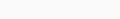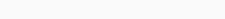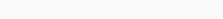# Coursera machine learning week 6 Quiz answers System Design | Andrew NG

In this article, you will find Coursera machine learning week 6 Quiz answers System Design | Andrew NG.  Use “Ctrl+F” To Find Any Questions or Answers. For Mobile Users, You Just Need To Click On Three dots In Your Browser & You Will Get A “Find” Option There. Use These Options to Get Any Random Questions Answer.
Try to solve all the assignments by yourself first, but if you get stuck somewhere then feel free to browse the code. Don’t just copy-paste the code for the sake of completion.  Even if you copy the code, make sure you understand the code first.

### Coursera machine learning week 6 Quiz answers System Design

1. You are working on a spam classification system using regularized logistic regression. “Spam” is a positive class (y = 1) and “not spam” is the negative class (y = 0). You have trained your classifier and there are m = 1000 examples in the cross-validation set. The chart of predicted class vs. actual class is:For reference:

Accuracy = (true positives + true negatives) / (total examples)
Precision = (true positives) / (true positives + false positives)
Recall = (true positives) / (true positives + false negatives)
F1 score = (2 * precision * recall) / (precision + recall)
What is the classifier’s F1 score (as a value from 0 to 1)?
Enter your answer in the box below. If necessary, provide at least two values after
the decimal point.

0.16
Precision is 0.087 and recall is 0.85, so F1 score is (2 * precision * recall) /
(precision + recall) = 0.158.

NOTE:
Accuracy = (85 + 10) / (1000) = 0.095
Precision = (85) / (85 + 890) = 0.087
Recall = There are 85 true positives and 15 false negatives, so recall is
85 / (85 + 15) = 0.85.
F1 Score = (2 * (0.087 * 0.85)) / (0.087 + 0.85) = 0.16

2. Suppose a massive dataset is available for training a learning algorithm. Training on a lot of data is likely to give good performance when two of the following conditions hold true.
Which are the two?

•  We train a learning algorithm with a large number of parameters (that is able to learn/represent fairly complex functions).

You should use a “low bias” algorithm with many parameters, as it will be able to make use of the large dataset provided. If the model has too few
parameters, it will underfit the large training set.

•  The features x contain sufficient information to predict accurately. (For example, one way to verify this is if a human expert on the domain can confidently predict when given only ).

It is important that the features contain sufficient information, as otherwise no
amount of data can solve a learning problem in which the features do not
contain enough information to make an accurate prediction.

•  We train a learning algorithm with a small number of parameters (that is thus unlikely to overfit).
•  We train a model that does not use regularization.

Even with a very large dataset, some regularization is still likely to help the algorithm’s performance, so you should use cross-validation to select the appropriate regularization parameter.

•  The classes are not too skewed.

The problem of skewed classes is unrelated to training with large datasets.

•  Our learning algorithm is able to represent fairly complex functions (for example, if we train a neural network or other model with a large number of parameters).

You should use a complex, “low bias” algorithm, as it will be able to make use of the large dataset provided. If the model is too simple, it will underfit the large training set.

•  A human expert on the application domain can confidently predict y when given only the features x (or more generally we have some way to be confident that x contains sufficient information to predict y accurately)

This is a nice project commencement briefing.

3. Suppose you have trained a logistic regression classifier which is outputing.
Currently, you predict 1 if, and predict 0 if, where currently the threshold is set to 0.5.
Suppose you increase the threshold to 0.9. Which of the following are true? Check all that apply.

•  The classifier is likely to have unchanged precision and recall, but higher accuracy.
•  The classifier is likely to now have higher recall.
•  The classifier is likely to now have higher precision.

Increasing the threshold means more y = 0 predictions. This will decrease both true and false positives, so precision will increase.

•  The classifier is likely to have unchanged precision and recall, and thus the same F1 score.
•  The classifier is likely to now have lower recall.

Increasing the threshold means more y = 0 predictions. This increase will decrease the number of true positives and increase the number of false negatives, so recall will decrease.

•  The classifier is likely to now have lower precision.

3. Suppose you have trained a logistic regression classifier which is outputing.
Currently, you predict 1 if, and predict 0 if, where currently the threshold is set to 0.5.
Suppose you decrease the threshold to 0.3. Which of the following are true? Check all that apply.

•  The classifier is likely to have unchanged precision and recall, but higher accuracy.
•  The classifier is likely to have unchanged precision and recall, but lower accuracy.
•  The classifier is likely to now have higher recall.

Recall = (true positives) / (true positives + false negatives)
Decreasing the threshold means less y = 0 predictions. This will increase true positives and decrease the number of false negatives, so recall will increase.

•  The classifier is likely to now have higher precision.
•  The classifier is likely to have unchanged precision and recall, and thus the same F1 score.
•  The classifier is likely to now have lower recall.
•  The classifier is likely to now have lower precision.

Lowering the threshold means more y = 1 predictions. This will increase both true and false positives, so precision will decrease.

4. Suppose you are working on a spam classifier, where spam emails are positive examples (y = 1) and non-spam emails are negative examples (y = 0). You have a training set of emails in which 99% of the emails are non-spam and the other 1% is spam.
Which of the following statements are true? Check all that apply.

•  A good classifier should have both a high precision and high recall on the cross validation set.

For data with skewed classes like these spam data, we want to achieve a
high F1 score, which requires high precision and high recall.

•  If you always predict non-spam (output y=0), your classifier will have an accuracy of 99%.

Since 99% of the examples are y = 0, always predicting 0 gives an accuracy of 99%. Note, however, that this is not a good spam system, as you will never catch any spam.

•  If you always predict non-spam (output y=0), your classifier will have 99% accuracy on the training set, but it will do much worse on the cross validation set because it has overfit the training data.

The classifier achieves 99% accuracy on the training set because of how skewed the classes are. We can expect that the cross-validation set will be skewed in the same fashion, so the classifier will have approximately the same accuracy.

•  If you always predict non-spam (output y=0), your classifier will have 99% accuracy on the training set, and it will likely perform similarly on the cross validation set.

The classifier achieves 99% accuracy on the training set because of how skewed the classes are. We can expect that the cross-validation set will be skewed in the same fashion, so the classifier will have approximately the same accuracy.

5. Which of the following statements are true? Check all that apply.

•  Using a very large training set makes it unlikely for model to overfit the training data.

A sufficiently large training set will not be overfit, as the model cannot overfit some of the examples without doing poorly on the others.

•  After training a logistic regression classifier, you must use 0.5 as your threshold for predicting whether an example is positive or negative.

You can and should adjust the threshold in logistic regression using cross validation data.

•  If your model is underfitting the training set, then obtaining more data is likely to help.

If the model is underfitting the training data, it has not captured the information in the examples you already have. Adding further examples will not help any more.

•  It is a good idea to spend a lot of time collecting a large amount of data before building your first version of a learning algorithm.

It is not recommended to spend a lot of time collecting a large data

•  On skewed datasets (e.g., when there are more positive examples than negative examples), accuracy is not a good measure of performance and you should instead use F1 score based on the precision and recall.

You can always achieve high accuracy on skewed datasets by predicting the most the same output (the most common one) for every input. Thus the F1 score is a better way to measure performance.

•  The “error analysis” process of manually examining the examples which your algorithm got wrong can help suggest what are good steps to take (e.g., developing new features) to improve your algorithm’s performance.

This process of error analysis is crucial in developing high performance learning systems, as the space of possible improvements to your system is very large, and it gives you direction about what to work on next.

Disclaimer:  Hopefully, this article will be useful for you to find all the Coursera machine learning week 6 Quiz answers System Design and grab some premium knowledge with less effort.
Finally, we are now, in the end, I just want to conclude some important message for you, Feel free to ask doubts in the comment section. I will try my best to answer it. If you find this helpful by any means like, comment, and share the post. Please share our posts on social media platforms and also suggest to your friends to Join Our Groups. Don’t forget to subscribe. This is the simplest way to encourage me to keep doing such work.

### FAQs

Is Andrew Ng’s Machine Learning course good?
It is the Best Course for Supervised Machine Learning! Andrew Ng Sir has been like always has such important & difficult concepts of Supervised ML with such ease and great examples, Just amazing!
How do I get answers to coursera assignment?
Use “Ctrl+F” To Find Any Questions Answered. & For Mobile Users, You Just Need To Click On Three dots In Your Browser & You Will Get A “Find” Option There. Use These Options to Get Any Random Questions Answer.
How long does it take to finish coursera Machine Learning?
this specialization requires approximately 3 months with 75 hours of materials to complete, and I finished it in 3 weeks and spent an additional 1 week reviewing the whole course.
How do you submit assignments on Coursera Machine Learning?
Submit a programming assignment Open the assignment page for the assignment you want to submit. Read the assignment instructions and download any starter files. Finish the coding tasks in your local coding environment. Check the starter files and instructions when you need to. Reference

Sharing Is Caring